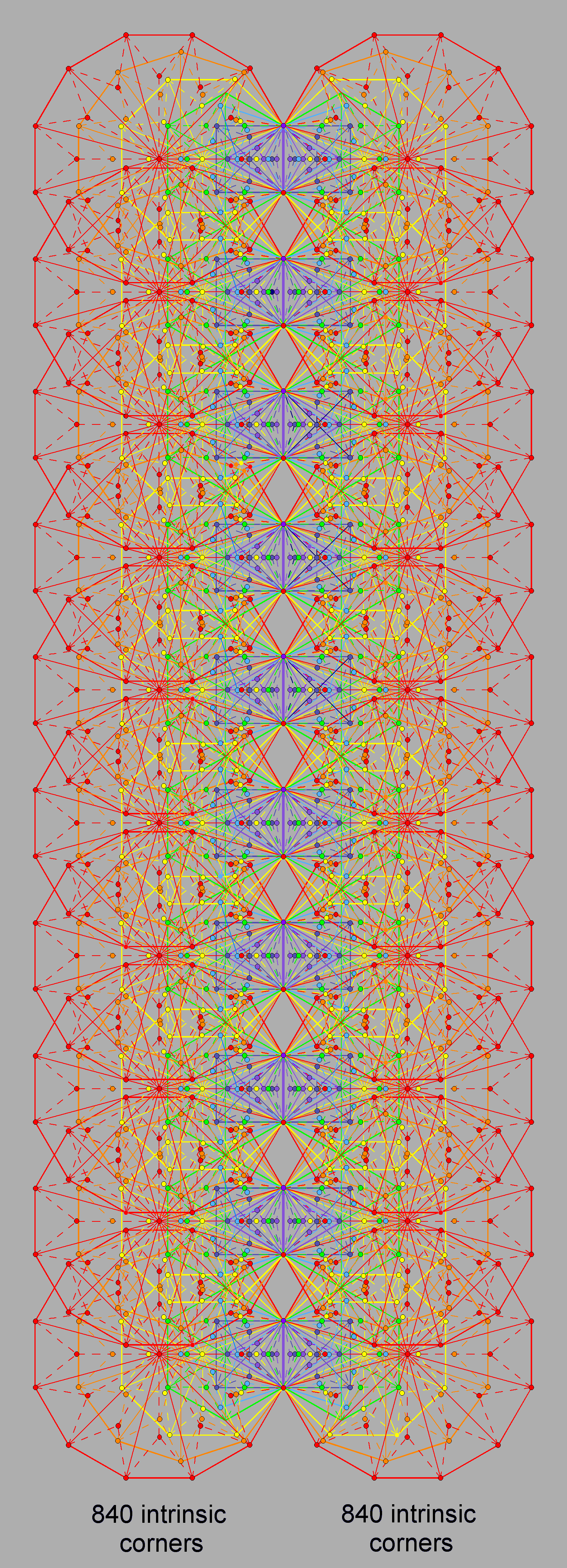<< Previous    1...   24  25    27  28  ...56    Next >>

#26 The Type B polygons in the inner form of ten Trees of Life embody the superstring structural parameter 1680The (7+7) enfolded Type A polygons have (47+47=94) sectors with 80 corners and 175 sides. When each sector is a Type A triangle, 94 corners and (3×94=282) sides are added to what are now (3×94=282) triangles. 282 is the number value of Aralim, the Order of Angels assigned to Binah in the Tree of Life. Hence, the 282 triangles in the (7+7) enfolded Type B polygons have (80+94=174) corners and (175+282=457) sides, i.e., there are 913 geometrical elements. The number of sides outside the root edge is equal to the number of corners & triangles.

Chokmah, Chesed & Netzach coincide with corners of the triangle and hexagon on the right-hand side and Binah, Geburah & Hod coincide with corners of the triangle and hexagon on the left-hand side. Two internal sides of the right-hand hexagon form the vertical Pillar of Mercy and two internal sides of the left-hand hexagon form the Pillar of Judgement. Six corners and four sides of triangles in the enfolded polygons, i.e., ten corners & sides, are shared with its outer form, leaving 900 (=90×10) geometrical elements outside the root edge that are intrinsic to the 14 enfolded polygons.

The number of intrinsic geometrical elements in each set and in both sets of the seven enfolded Type B polygons is shown below:

 Corners Sides Triangles Total 7 enfolded polygons 85 227 141 453 (7+7) enfolded polygons 168 453 282 903

The 141 triangles in the seven enfolded Type B polygons have 85 intrinsic corners, where

85 = 40 + 41 + 42 + 43.

This is the number of yods in the 2nd-order tetractys (see here), demonstrating the holistic nature of the seven enfolded polygons. Remarkably, the powers of 4 denote the numbers of different sets of corners. 40 = 1 denotes the upper endpoint (the start of the generation of the polygons), 41 = 4 is the number of intrinsic corners inside the triangle, 42 = 16 is the number of intrinsic corners in the square & pentagon and 43 = 64 is the number of intrinsic corners in the hexagon, octagon, decagon & dodecagon (see also #44 in Wonders of sacred geometry/correspondences).

The table above indicates that the 282 triangles making up the (7+7) enfolded Type B polygons have 168 intrinsic corners, 84 such corners being associated with each set of seven polygons. 168 is the number value of Cholem Yesodoth, the Mundane Chakra of Malkuth. There are 2820 triangles in the (70+70) Type B polygons enfolded in the ten overlapping Trees of Life that can represent the ten Sephiroth of a single Tree of Life. They have 1680 intrinsic corners, 840 corners being associated with each set of 70 polygons. This constitutes a truly remarkable, geometrical encoding of the 1680 turns of the helical whorl in the UPA described by C.W. Leadbeater. Each comer corresponds to a turn in the helical whorl (see here), and the two halves of the inner form of ten overlapping Trees of Life correspond to the outer and inner halves of each whorl. Here is powerful evidence that his ability to magnify subatomic particles with the yogic siddhi of anima was genuine. Just as the 1680 corners of the triangles shape the inner form of the ten Trees of Life, so, too, the 1680 circularly polarized oscillations of each whorl create its helical form as a standing wave. The fundamental subquark state of the E8×E8 heterotic superstring is the physical realization of the sacred geometrical blueprint of the Tree of Life.

 << Previous    1...   24  25    27  28  ...56    Next >>

Home